# Heterogeneity & Demographic Analysis

## Introduction

Heterogeneity analysis is a way to explore how the results of a model can vary depending on the characteristics of individuals in a population, and demographic analysis estimates the average values of a model over an entire population.

In practice these two analyses naturally complement each other: heterogeneity analysis runs the model on multiple sets of parameters (reflecting differents characteristics found in the target population), and demographic analysis combines the results.

For this example we will use the result from the assessment of a new total hip replacement previously described in vignette("d-non-homogeneous", "heemod").

## Population characteristics

The characteristics of the population are input from a table, with one column per parameter and one row per individual. Those may be for example the characteristics of the indiviuals included in the original trial data.

For this example we will use the characteristics of 100 individuals, with varying sex and age, specified in the data frame tab_indiv:

tab_indiv
## # A tibble: 100 x 2
##      age   sex
##    <dbl> <int>
##  1    64     1
##  2    49     0
##  3    77     0
##  4    55     1
##  5    72     1
##  6    50     0
##  7    56     0
##  8    50     0
##  9    63     0
## 10    50     0
## # … with 90 more rows
library(ggplot2)
ggplot(tab_indiv, aes(x = age)) +
geom_histogram(binwidth = 2)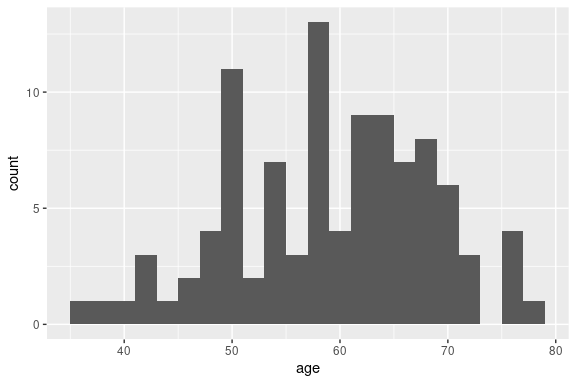## Running the analysis

res_mod, the result we obtained from run_model() in the Time-varying Markov models vignette, can be passed to update() to update the model with the new data and perform the heterogeneity analysis.

res_h <- update(res_mod, newdata = tab_indiv)
## No weights specified in update, using equal weights.
## Updating strategy 'standard'...
## Updating strategy 'np1'...

## Interpreting results

The summary() method reports summary statistics for cost, effect and ICER, as well as the result from the combined model.

summary(res_h)
## An analysis re-run on 100 parameter sets.
##
## * Unweighted analysis.
##
## * Values distribution:
##
##                                  Min.      1st Qu.      Median        Mean
## standard - Cost          530.94590166  605.0062810 631.4705169 700.1739521
## standard - Effect         17.56922957   25.5696426  27.3769142  26.4351997
## standard - Cost Diff.               -            -           -           -
## standard - Effect Diff.             -            -           -           -
## standard - Icer                     -            -           -           -
## np1 - Cost               615.48340627  635.5509751 642.7469056 662.6400050
## np1 - Effect              17.66126700   25.8299343  27.7656911  26.7056482
## np1 - Cost Diff.        -160.47985885 -129.4829089  11.2763887 -37.5339471
## np1 - Effect Diff.         0.09203743    0.1948185   0.2214442   0.2704486
## np1 - Icer              -352.23489020 -333.0519971  47.6378220 -30.7355758
##                             3rd Qu.        Max.
## standard - Cost         828.5434528 871.8854128
## standard - Effect        29.0749005  31.5986556
## standard - Cost Diff.             -           -
## standard - Effect Diff.           -           -
## standard - Icer                   -           -
## np1 - Cost              699.0605439 711.4055539
## np1 - Effect             29.5008365  31.8353665
## np1 - Cost Diff.         30.5446941  84.5375046
## np1 - Effect Diff.        0.3887769   0.4556047
## np1 - Icer              156.7853582 918.5122572
##
## * Combined result:
##
## 2 strategies run for 60 cycles.
##
## Initial state counts:
##
## PrimaryTHR = 1000L
## SuccessP = 0L
## RevisionTHR = 0L
## SuccessR = 0L
## Death = 0L
##
## Counting method: 'beginning'.
##
## Values:
##
##           utility   cost
## standard 26435.20 700174
## np1      26705.65 662640
##
## Efficiency frontier:
##
## np1
##
## Differences:
##
##     Cost Diff. Effect Diff.      ICER     Ref.
## np1  -37.53395    0.2704486 -138.7841 standard

The variation of cost or effect can then be plotted.

plot(res_h, result = "effect", binwidth = 5)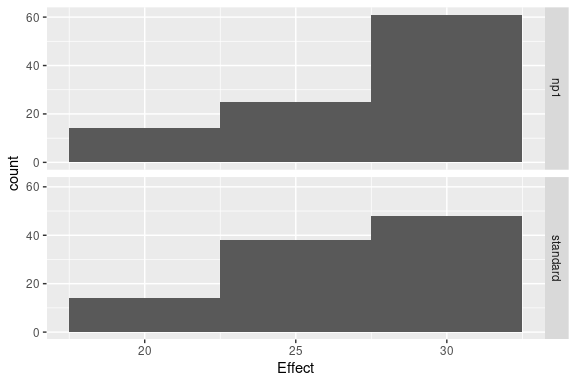plot(res_h, result = "cost", binwidth = 50)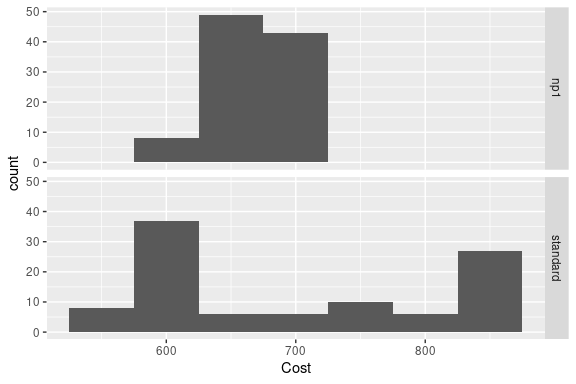plot(res_h, result = "icer", type = "difference",
binwidth = 500)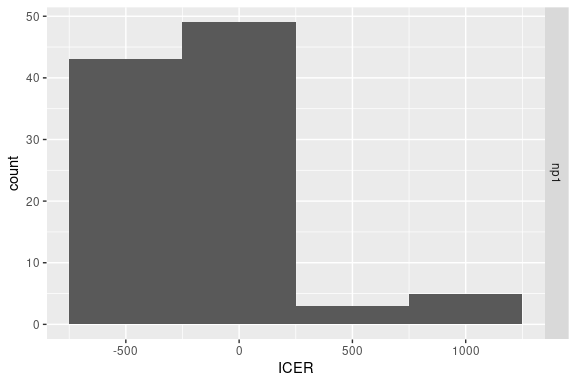plot(res_h, result = "effect", type = "difference",
binwidth = .1)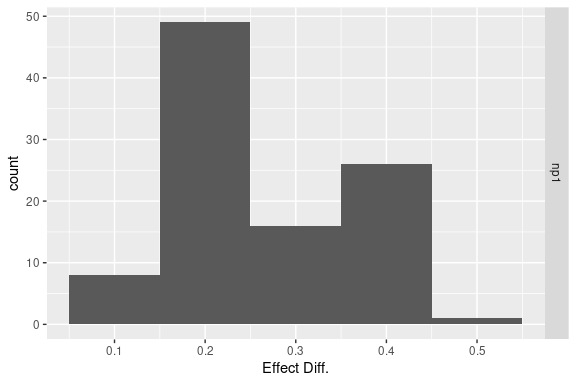plot(res_h, result = "cost", type = "difference",
binwidth = 30)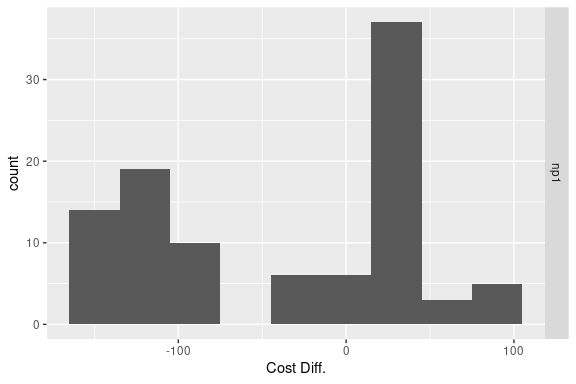The results from the combined model can be plotted similarly to the results from run_model().

plot(res_h, type = "counts")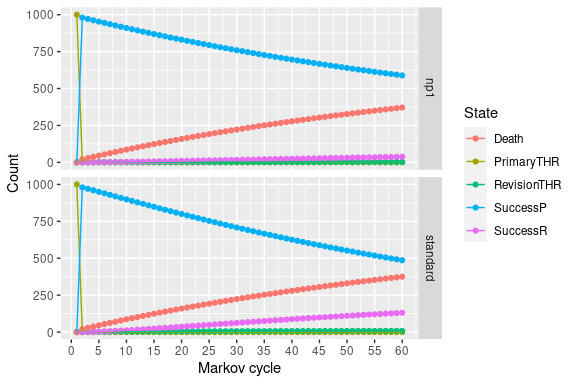## Weighted results

Weights can be used in the analysis by including an optional column .weights in the new data to specify the respective weights of each strata in the target population.

tab_indiv_w
res_w <- update(res_mod, newdata = tab_indiv_w)
res_w

## Parallel computing

Updating can be significantly sped up by using parallel computing. This can be done in the following way:

• Define a cluster with the use_cluster() functions (i.e. use_cluster(4) to use 4 cores).
• Run the analysis as usual.
• To stop using parallel computing use the close_cluster() function.

Results may vary depending on the machine, but we found speed gains to be quite limited beyond 4 cores.